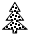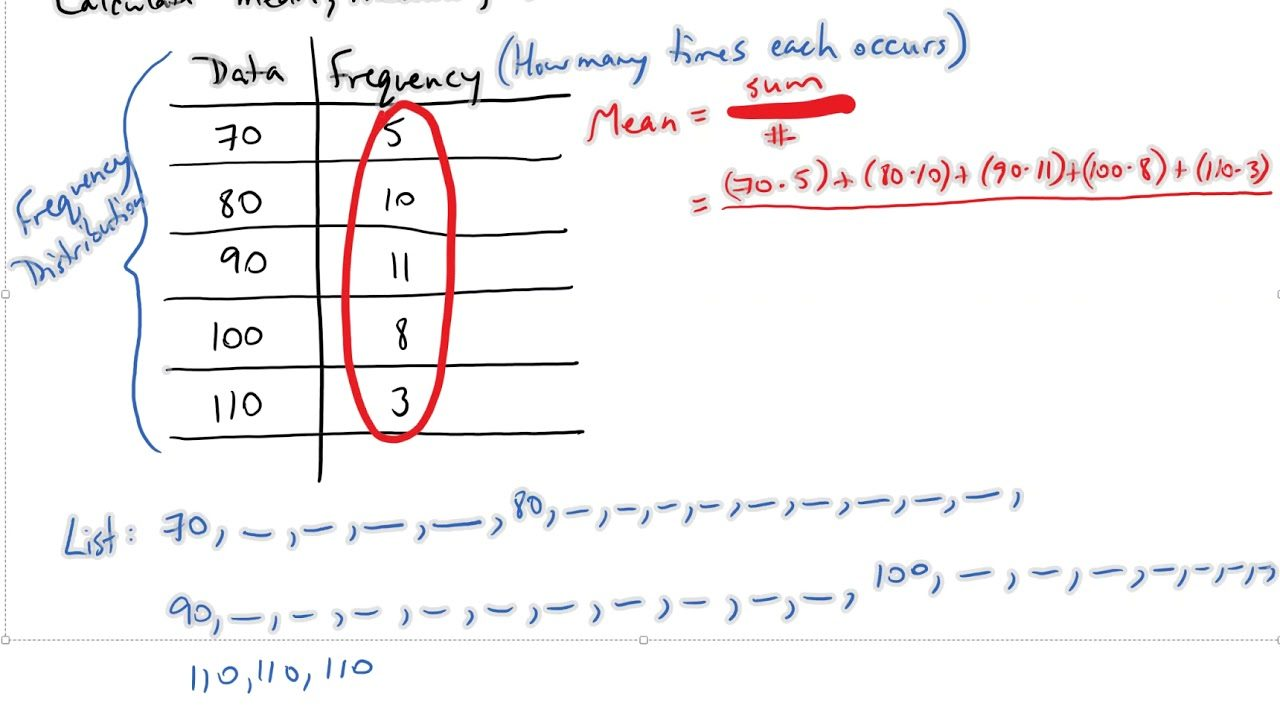# Graphs, range, meridian and mean P.4

##### This unit is about Graphs, mean among others.

GRAPHS

Reading and interpreting picture / pictographs

The pictograph below show the number of trees planted by three boys.

 OkotOkelloKintuScale:=        5 trees

1. a) How many trees were planted by Kintu?

=        5 x 5

=        25 trees

1. b) How many more trees were planted by Okot than Okello?

Okot  >        3 x 5  =        15 trees

Okello>       2 x 5  =        10 trees

More trees   >                 1 5 trees

1 0 trees

0 5 more trees

1. c) Find the total number of trees planted.

=        10 x 5

=        50 trees

______________________________________________________________________

Drawing pictographs

Make a pictographs for the following information.

• John has 12 balls
• Mega has 15 balls
• Sarah has 18 balls
• Kamoga has 9 balls.

Scale:=        3 balls

Solution:

John > 12 = 4m

3                3

Mega > 15 =        5 balls

3

Sarah          > 18 =        6  balls

3

Kamoga> 9 =        3 balls

3

 NAME NUMBER OF BALLS JohnMegaSarahKamoga______________________________________________________________________

BAR GRAPHS

Interpreting the scales of a bar graph

The bar graph below shows the daily attendance of P4 pupils for a week. Study it and answers the following questions.a) What is represented on the vertical axis?

b) What is represented on the horizontal axis?

c) How many pupils were present on Thursday?

d) Which days had the same number of attendance?

e) How many more pupils attended on Wednesday than Friday?

Tallies

Organizing data by tallying

Example 1

One 1

Two  11

Three  111

Five     1111

Ten     1111  1111

Twelve  1111  1111  11

Finding the mode

Mode is the term or the number that appears many times.

Example 1

Kennedy got the following marks in an exam 70,88,70,90,85.

Find his modal mark

 MARK TALLY 70 11 85 1 88 1 90 1

Therefore the mode is 70

Finding the range

Range is the difference between the highest and the smallest.

Maureen scored the following goal in the four netball matches

7,9,6,5.

Find the range.

9-5 =4

FINDING THE MEDIAN

Median is the middle term or number.

It’s got by arranging the terms or number in ascending or descending order.

example

Find the median of ;

43,74,49,90,10.

10,43.49,74,90.

There for  the median is 49

FINDING THE MEAN,

Mean is total sum of given marks divided by the total number.

Example;

Find the mean of the following numbers.

3,5,4,6,2.

Mean    = 2+3+4+5+6

5

20

5

SEE ALL
•YOU